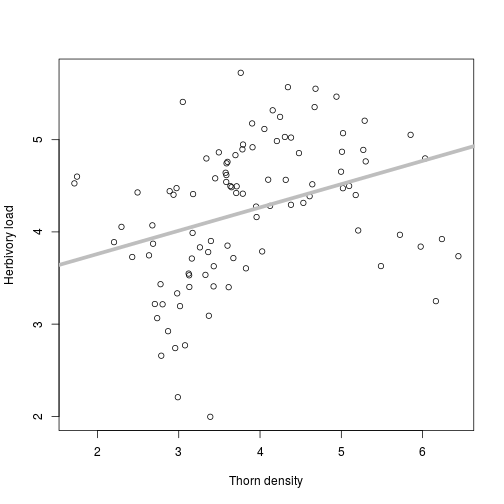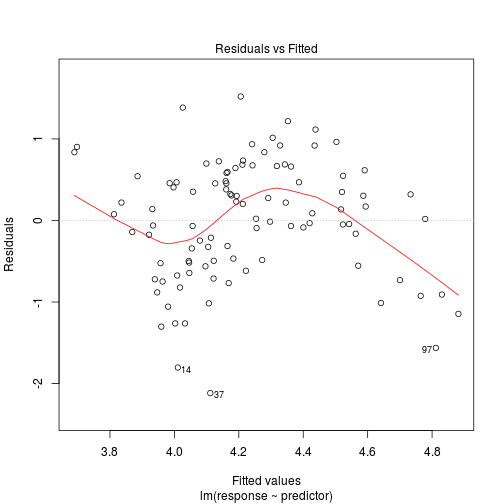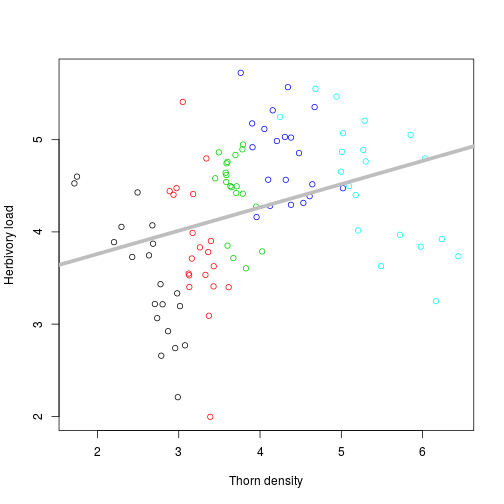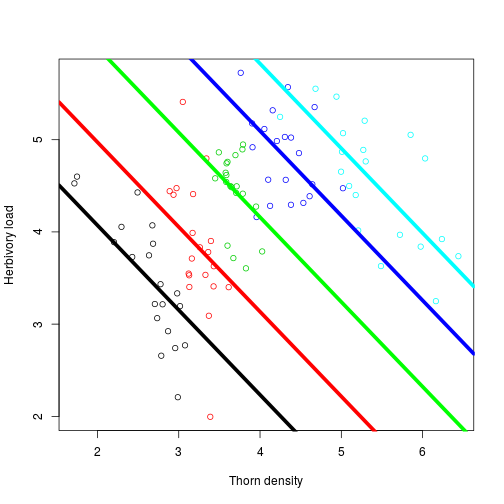# Introduction to linear mixed models

timotheenivalis.github.io
June 15 2018

### Example: hidden relationships

thorns <- read.table(file = "thorndata.txt", header=TRUE)

plot(thorns$response, x=thorns$predictor, ylab = "Herbivory load", xlab= "Thorn density")
abline(lm(response~ predictor, data=thorns), lwd=5, col="gray")#this is a shortcut to draw a regression line### Example: hidden relationships

lmthorns <- lm(response~ predictor, data=thorns)
summary(lmthorns)


Call:
lm(formula = response ~ predictor, data = thorns)

Residuals:
Min       1Q   Median       3Q      Max
-2.11617 -0.51831  0.08215  0.54349  1.51932

Coefficients:
Estimate Std. Error t value Pr(>|t|)
(Intercept)   3.2564     0.2854   11.41  < 2e-16 ***
predictor     0.2524     0.0717    3.52 0.000657 ***
---
Signif. codes:  0 '***' 0.001 '**' 0.01 '*' 0.05 '.' 0.1 ' ' 1

Residual standard error: 0.7252 on 98 degrees of freedom
Multiple R-squared:  0.1122,    Adjusted R-squared:  0.1032
F-statistic: 12.39 on 1 and 98 DF,  p-value: 0.0006566


### Example: hidden relationships

plot(lmthorns, which=1)plot(thorns$predictor, thorns$response, col=thorns$block, ylab = "Herbivory load", xlab= "Thorn density") abline(lm(response~ predictor, data=thorns), lwd=5, col="gray")### Example: hidden relationships Fixed-effect correction summary(lm(response~ predictor + as.factor(block), data=thorns))   Call: lm(formula = response ~ predictor + as.factor(block), data = thorns) Residuals: Min 1Q Median 3Q Max -1.68769 -0.28889 0.04982 0.24924 1.39683 Coefficients: Estimate Std. Error t value Pr(>|t|) (Intercept) 6.0471 0.3872 15.617 < 2e-16 *** predictor -0.9752 0.1414 -6.899 6.02e-10 *** as.factor(block)2 0.9400 0.1762 5.334 6.62e-07 *** as.factor(block)3 1.9958 0.2147 9.295 5.80e-15 *** as.factor(block)4 2.9706 0.2812 10.562 < 2e-16 *** as.factor(block)5 3.7674 0.4219 8.929 3.48e-14 *** --- Signif. codes: 0 '***' 0.001 '**' 0.01 '*' 0.05 '.' 0.1 ' ' 1 Residual standard error: 0.4875 on 94 degrees of freedom Multiple R-squared: 0.6151, Adjusted R-squared: 0.5947 F-statistic: 30.05 on 5 and 94 DF, p-value: < 2.2e-16  ### Example: hidden relationships library(lme4) thornLMM <- lmer(response ~ predictor + (1|block), data = thorns) summary(thornLMM)  Linear mixed model fit by REML ['lmerMod'] Formula: response ~ predictor + (1 | block) Data: thorns REML criterion at convergence: 165.3 Scaled residuals: Min 1Q Median 3Q Max -3.4884 -0.6059 0.1091 0.5234 2.8735 Random effects: Groups Name Variance Std.Dev. block (Intercept) 2.129 1.4590 Residual 0.238 0.4878 Number of obs: 100, groups: block, 5 Fixed effects: Estimate Std. Error t value (Intercept) 7.7652 0.8441 9.199 predictor -0.9189 0.1385 -6.633 Correlation of Fixed Effects: (Intr) predictor -0.632  ### Example: hidden relationships### Exercise: more hidden relationships Load the data thornsmanylocations.txt Compare lm() and lmer() corrections for block. ## What are random effects? ### Residuals and random effects Assumed variance-covariance matrix of the process that generates the residuals obs1 obs2 obs3 obs4 obs5 obs1 1 0 0 0 0 obs2 0 1 0 0 0 obs3 0 0 1 0 0 obs4 0 0 0 1 0 obs5 0 0 0 0 1 residuals are perfectly correlated with themselves, and independent of each-other ### Residuals and random effects If multiple measurements: obs1 obs2 obs3 obs4 obs5 ind1 ind1 ind2 ind2 ind3 obs1 ind1 1 1 0 0 0 obs2 ind1 1 1 0 0 0 obs3 ind2 0 0 1 1 0 obs4 ind2 0 0 1 1 0 obs5 ind3 0 0 0 0 1 among individuals, residuals are correlated with each-other ### Residuals and random effects Individual var-cov ind1 ind2 ind3 ind1 1 0 0 ind2 0 1 0 ind3 0 0 1 Residual var-cov obs1 obs2 obs3 obs4 obs5 obs1 1 0 0 0 0 obs2 0 1 0 0 0 obs3 0 0 1 0 0 obs4 0 0 0 1 0 obs5 0 0 0 0 1 ### NB: phylogenies, spatial correlation, time-series, genetic similarity... …are mixed models with correlations across random effect levels (individuals, species, locations…) obs1 obs2 obs3 obs4 obs5 ind1 ind1 ind2 ind3 ind4 obs1 ind1 1 1 0.25 0 0.01 obs2 ind1 1 1 0 0 0 obs3 ind2 0.25 0 1 0 0 obs4 ind3 0 0 0 1 0.125 obs5 ind4 0.01 0 0 0.125 1 Correlations represent (co-)variation that is unexplained, but is related to a biological process ### Residuals and random effects $y_{ij} = \mu + \beta x_{ij} + u_i + \epsilon_{ij}$ with, residuals $$\epsilon_{ij}\sim Normal(0,V_R)$$ and individual random effect $$u_{i}\sim Normal(0,V_I)$$. Random effects are cool because: • More efficient than estimating many independent fixed effects • Avoid distration from many coefficients and p-values • Test effect of grouping variable as one parameter • Variance components biologically interesting (e.g. repeatability $$V_I/(V_I+V_R)$$) ## Testing random effects significance ### Likelihood Ratio Test (LRT) Comparison of two nested models. Ratio of likelihood $$\sim \chi^2$$ thornLMM <- lmer(response ~ predictor + (1|block), data = thorns) thornLM <- lm(response ~ predictor, data = thorns) anova(thornLMM, thornLM) # the mixed model MUST GO FIRST  Data: thorns Models: thornLM: response ~ predictor thornLMM: response ~ predictor + (1 | block) Df AIC BIC logLik deviance Chisq Chi Df Pr(>Chisq) thornLM 3 223.50 231.31 -108.749 217.50 thornLMM 4 172.05 182.47 -82.027 164.05 53.445 1 2.66e-13 *** --- Signif. codes: 0 '***' 0.001 '**' 0.01 '*' 0.05 '.' 0.1 ' ' 1  ### Exercise: LRT p-value distribution One simulation with a random effect that has no effect set.seed(1234) RandomVariance <- 0 sampsize <- 200 x <- rnorm(sampsize,mean = 4, sd=0.25) nbblocks <- 50 block <- sample(x = 1:nbblocks, size = sampsize, replace = TRUE) blockvalues <- rnorm(n = nbblocks, mean = 0, sd = sqrt(RandomVariance)) y <- 8 - x + blockvalues[block] + rnorm(sampsize,0,1) dat <- data.frame(response = y, predictor = x, block=block)  lm0 <- lm(response ~ 1 + predictor, data=dat) lmm0 <- lmer(response ~ 1 + predictor + (1|block), data=dat ) (LRT0 <- anova(lmm0, lm0)) #mixed model must come first! LRT0$Pr(>Chisq) # the p-value


### Exercise: LRT p-value distribution

Replicate the simulations to obtain the distribution of p-values under the null-model of no variance

### Correct p-values in LRT

A variance cannot be negative

confint(lmm0) #Confidence interval


LRT are two sided tests / count one parameter per random effect A random effect is half a parameter / to be tested with one-side tests

Divide the p-values by two

Same problem with AIC/BIC: count only half a parameter per random effect Remove one IC point per random effect

NB: it is more complicated with random interactions; but the rule is to count half a parameter by variance parameter

### Test / remove non-significant random effects?

Test ?

• Yes if effect of interest
• Maybe if only a nuisance parameter

Remove ?

• Probably should keep if part of exp. design
• Doesn't matter much if non-significant
• Maybe remove if too many variables in exploratory analyses